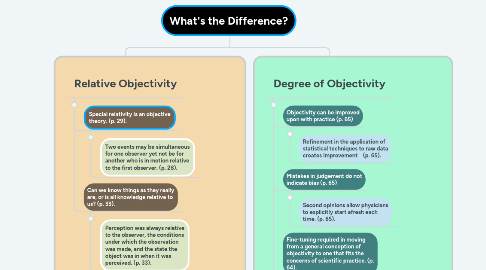# What's the Difference?Ross Tulman
Get Started. It's FreeWhat's the Difference?## 1. Relative Objectivity

### 1.1. Special relativity is an objective theory. (p. 29).

1.1.1. Two events may be simultaneous for one observer yet not be for another who is in motion relative to the first observer. (p. 28).

### 1.2. Can we know things as they really are, or is all knowledge relative to us? (p. 33).

1.2.1. Perception was always relative to the observer, the conditions under which the observation was made, and the state the object was in when it was perceived. (p. 33).

1.2.2. Everything we thought to be absolute turns out to be relative. (p. 36).

1.2.2.1. There can be no account of the world that doesn’t involve some assumptions and values, and hence prejudice or bias, and therefore there can be no objective account of the world. (p. 36).

1.2.2.1.1. Conclusion: there can be legitimate dispute about what is relative and what is absolute. We may decide that something which we had considered to be absolute turns out to be relative. (p. 36).

## 2. Degree of Objectivity

### 2.1. Objectivity can be improved upon with practice (p. 65)

2.1.1. Refinement in the application of statistical techniques to raw data creates improvement (p. 65).

### 2.2. Mistakes in judgement do not indicate bias (p. 65)

2.2.1. Second opinions allow physicians to explicitly start afresh each time. (p. 65).

### 2.3. Fine-tuning required in moving from a general conception of objectivity to one that fits the concerns of scientific practice. (p. 64).

2.3.1. An increase in accuracy of representation is correlated with an increase in proximity to the truth. (p. 66).

2.3.2. Conclusion: whereas truth is absolute and does not come in degrees, objectivity only comes in degrees. (p. 66)

2.3.2.1. Page Citations: Gaukroger, Stephen. Objectivity: a Very Short Introduction. Oxford University Press, 2012.